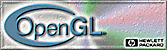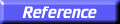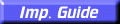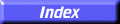# glFrustum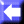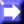glFrustum: multiply the current matrix by a perspective matrix.

C Specification | Parameters | Description | Notes | Errors | Associated Gets | See Also

##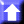C Specification

```void glFrustum(
GLdouble	 left,
GLdouble	 right,
GLdouble	 bottom,
GLdouble	 top,
GLdouble	 zNear,
GLdouble	 zFar)
```

##Parameters

left, right
Specify the coordinates for the left and right vertical clipping planes.
bottom, top
Specify the coordinates for the bottom and top horizontal clipping planes.
zNear, zFar
Specify the distances to the near and far depth clipping planes. Both distances must be positive.

##Description

glFrustum describes a perspective matrix that produces a perspective projection. The current matrix (see glMatrixMode) is multiplied by this matrix and the result replaces the current matrix, as if glMultMatrix were called with the following matrix as its argument:

 E 0 A 0 0 F B 0 0 0 C D 0 0
1 0
where:
A = (right + left) / (rightleft)
B = (top + bottom) / (topbottom)
C =(far + near) / (farnear)
D =(2
farnear) / (farnear)
E = (2near) / (rightleft)
F = (2near) / (topbottom)

Typically, the matrix mode is GL_PROJECTION, and (left, bottom,zNear) and (right, top,zNear) specify the points on the near clipping plane that are mapped to the lower left and upper right corners of the window, assuming that the eye is located at (0, 0, 0).zFar specifies the location of the far clipping plane. Both zNear and zFar must be positive.

Use glPushMatrix and glPopMatrix to save and restore the current matrix stack.

##Notes

Depth buffer precision is affected by the values specified for zNear and zFar. The greater the ratio of zFar to zNear is, the less effective the depth buffer will be at distinguishing between surfaces that are near each other. If

r = zFar / zNear

roughly log2 r bits of depth-buffer precision are lost. Because r approaches infinity as zNear approaches 0, zNear must never be set to 0.

##Errors

• GL_INVALID_VALUE is generated if zNear or zFar is not positive.
• GL_INVALID_OPERATION is generated if glFrustum is executed between the execution of glBegin and the corresponding execution of glEnd.

##Associated Gets

glGet with argument GL_MATRIX_MODE
glGet with argument GL_MODELVIEW_MATRIX
glGet with argument GL_PROJECTION_MATRIX
glGet with argument GL_TEXTURE_MATRIX

glOrtho,
glMatrixMode,
glMultMatrix,
glPushMatrix,
glViewport